# Example of a sunspot

The sunspot data is constituted of several dates assumed to be contemporaneous of a single event. These dates do not need any calibration but their unit is in year before 2016.

# Simple model for combining Gaussian dates

Let's first use a simple Bayesian model.

`````` library(ArchaeoChron)
data(sunspot)
MCMC1 = combination_Gauss(M=sunspot\$Age[1:5], s= sunspot\$Error[1:5], refYear=rep(2016,5), studyPeriodMin=900, studyPeriodMax=1500, variable.names = c('theta'))
``````
``````## Compiling model graph
##    Resolving undeclared variables
##    Allocating nodes
## Graph information:
##    Observed stochastic nodes: 5
##    Unobserved stochastic nodes: 1
##    Total graph size: 29
##
## Initializing model
##
##  "Update period"
##  "Acquire period"
``````

The output of the function combination_Gauss() is a Markov chain of the posterior distribution of the Bayesian model.

## Convergence of the Markov chain

First, let's check the convergence of the Markov chain of each parameter.

``````   library(coda)
plot(MCMC1)
``````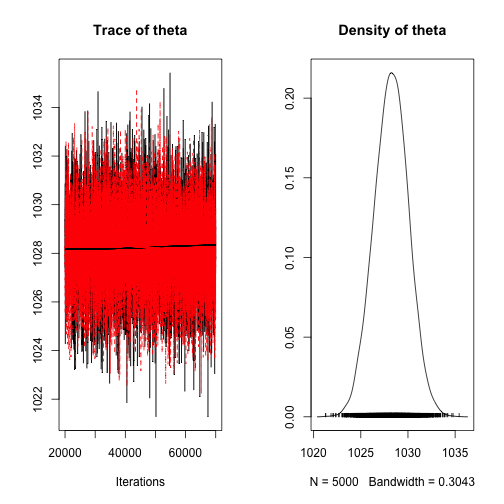``````   gelman.diag(MCMC1)
``````
``````## Potential scale reduction factors:
##
##       Point est. Upper C.I.
## theta          1          1
``````

The Gelman diagnotic gives point estimates about 1, so convergence is reached.

## Statistics of the posterior distribution of the event.

Now, we can use the package ArchaeoPhases in order to describe the posterior distribution of the parameters.

Here we will focus on the posterior distribution of the date of interest i.e. the parameter 'theta'.

``````   library(ArchaeoPhases)
MCMCSample1 = rbind(MCMC1[], MCMC1[])
M1 = MarginalStatistics(MCMCSample1[,1], level = 0.95)
M1
``````
``````##                         [,1]
## mean                 1028.00
## MAP                  1028.00
## sd                      2.00
## Q1                   1027.00
## median               1028.00
## Q2                   1029.00
## level                   0.95
## CredibleInterval Inf 1025.00
## CredibleInterval Sup 1032.00
## HPDRInf 1            1025.00
## HPRDSup 1            1032.00
``````
``````   MarginalPlot(MCMCSample1[,1], level = 0.95)
``````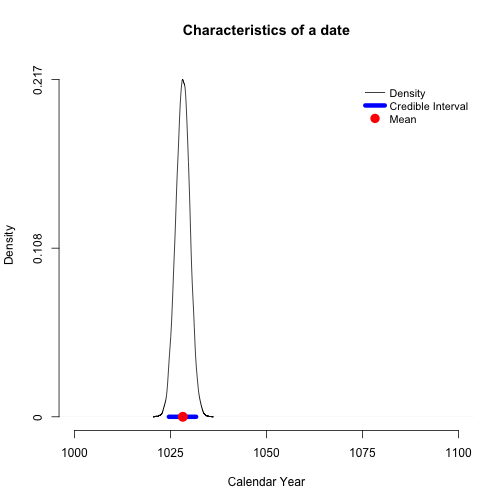The date (mean posterior distribution) of the sunspot estimated using a simple Bayesian model that combines dates, is dates at 1028 years after Christ. This date is associated with a 95% confidence interval : [1025, 1032].

# Bayesian model for combining Gaussian dates and handling potential outliers

Let's use the function combinationWithOutliers_Gauss().

``````    MCMC2 = combinationWithOutliers_Gauss(M=sunspot\$Age[1:5], s= sunspot\$Error[1:5], refYear=rep(2016,5), outliersIndivVariance = rep(1,5), outliersBernouilliProba=rep(0.2, 5), studyPeriodMin=800, studyPeriodMax=1500, variable.names = c('theta'))
``````
``````## Compiling model graph
##    Resolving undeclared variables
##    Allocating nodes
## Graph information:
##    Observed stochastic nodes: 5
##    Unobserved stochastic nodes: 11
##    Total graph size: 70
##
## Initializing model
##
##  "Update period"
##  "Acquire period"
``````
``````    plot(MCMC2)
``````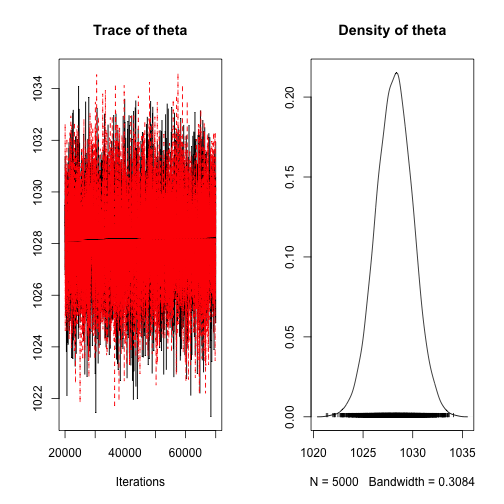The output of the function combinationWithOutliers_Gauss() is a Markov chain of the posterior distribution of the Bayesian model.

Here we will focus on the posterior distribution of the date of interest i.e. the parameter 'theta'.

``````   MCMCSample2 = rbind(MCMC2[], MCMC2[])
M2 = MarginalStatistics(MCMCSample2[,1], level = 0.95)
M2
``````
``````##                         [,1]
## mean                 1028.00
## MAP                  1028.00
## sd                      2.00
## Q1                   1027.00
## median               1028.00
## Q2                   1029.00
## level                   0.95
## CredibleInterval Inf 1025.00
## CredibleInterval Sup 1032.00
## HPDRInf 1            1025.00
## HPRDSup 1            1032.00
``````
``````   MarginalPlot(MCMCSample2[,1], level = 0.95)
``````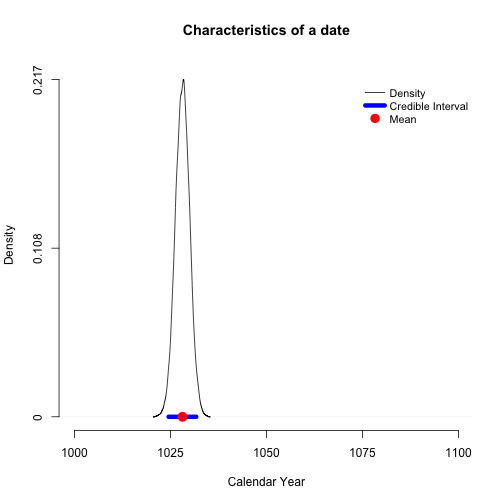The date (mean posterior distribution) of the sunspot, estimated using a simple Bayesian model that combines dates and allows for outliers, is dates at 1028 years after Christ. This date is associated with a 95% confidence interval : [1025, 1032].

# Bayesian model for combining Gaussian dates with a random effect

Let's now use the function combinationWithRandomEffect_Gauss().

``````    MCMC3 = combinationWithRandomEffect_Gauss(M=sunspot\$Age[1:5], s= sunspot\$Error[1:5], refYear=rep(2016,5), studyPeriodMin=0, studyPeriodMax=1500, variable.names = c('theta'))
``````
``````## Compiling model graph
##    Resolving undeclared variables
##    Allocating nodes
## Graph information:
##    Observed stochastic nodes: 5
##    Unobserved stochastic nodes: 7
##    Total graph size: 51
##
## Initializing model
##
##  "Update period"
##  "Acquire period"
``````
``````    plot(MCMC3)
``````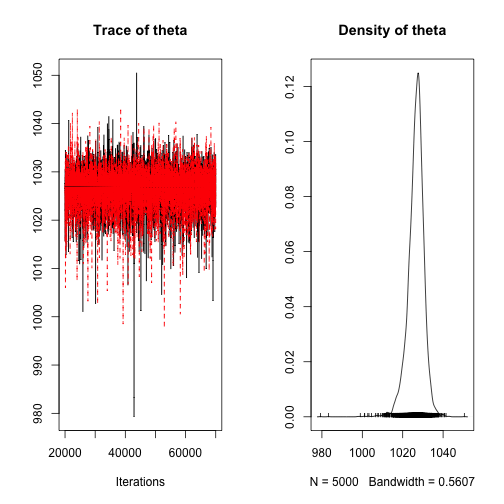The output of the function combinationWithRandomEffect_Gauss() is a Markov chain of the posterior distribution of the Bayesian model.

Here we will focus on the posterior distribution of the date of interest i.e. the parameter 'theta'.

``````   MCMCSample3 = rbind(MCMC3[], MCMC3[])
M3 = MarginalStatistics(MCMCSample3[,1], level = 0.95)
M3
``````
``````##                         [,1]
## mean                 1027.00
## MAP                  1027.00
## sd                      4.00
## Q1                   1024.00
## median               1027.00
## Q2                   1029.00
## level                   0.95
## CredibleInterval Inf 1019.00
## CredibleInterval Sup 1034.00
## HPDRInf 1            1018.00
## HPRDSup 1            1034.00
``````
``````   MarginalPlot(MCMCSample3[,1], level = 0.95)
``````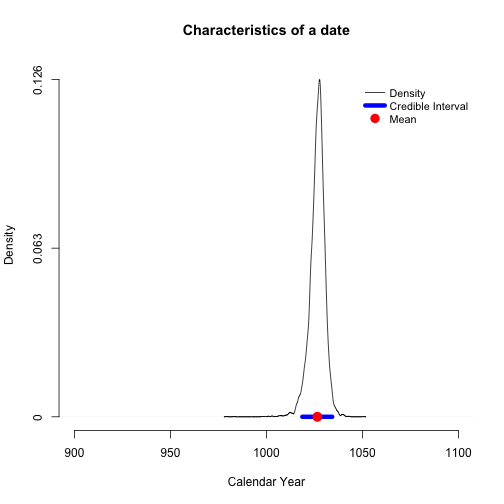The date (mean posterior distribution) of the sunspot, estimated using a simple Bayesian model that combines dates and allows for random effects, is dates at 1027 years after Christ. This date is associated with a 95% confidence interval : [1019, 1034].

# Event Model : Bayesian model for combining Gaussian dates with individual random effects

If we want to date that event, we can use the Event model for combining Gaussian dates. In that example, we will investigate the posterior distribution of the date of the event (called 'theta') and the posterior distribution of the dates associated with this event.

### Generating the Markov chain of the posterior distribution

Finally, let's use the function “eventModel_Gauss” and the first 10 dates of the dataset sunspot. The study period should be given in calendar year (BC/AD).

``````    MCMC4 = eventModel_Gauss(M=sunspot\$Age[1:5], s= sunspot\$Error[1:5], refYear=rep(2016,5), studyPeriodMin=900, studyPeriodMax=1500, variable.names = c('theta', 'mu'))
``````
``````## Compiling model graph
##    Resolving undeclared variables
##    Allocating nodes
## Graph information:
##    Observed stochastic nodes: 5
##    Unobserved stochastic nodes: 11
##    Total graph size: 111
##
## Initializing model
##
##  "Update period"
##  "Acquire period"
``````

The output of the “eventModel_Gauss” is the Markov chain of the posterior distribution.

### Convergence of the Markov chain

First, let's check the convergence of the Markov chain of each parameter.

``````   plot(MCMC4)
``````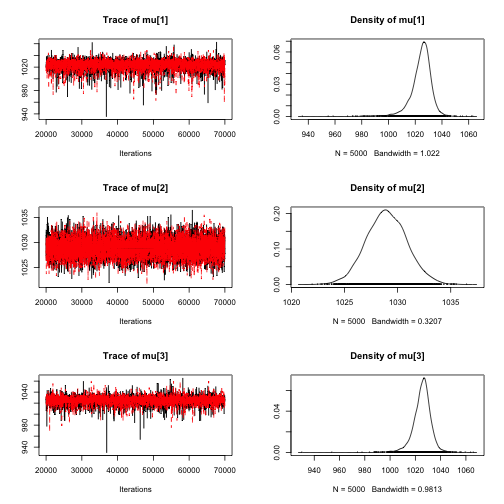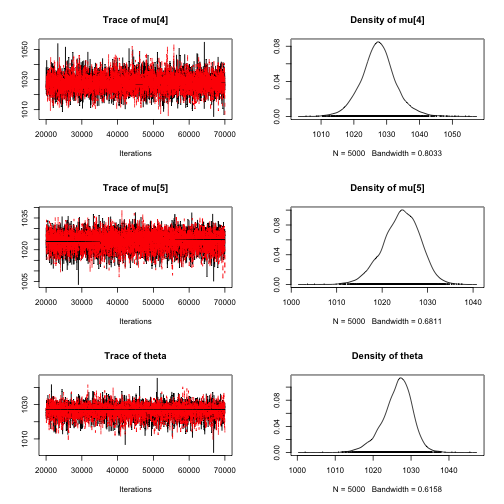``````   gelman.diag(MCMC4)
``````
``````## Potential scale reduction factors:
##
##       Point est. Upper C.I.
## mu          1          1
## mu          1          1
## mu          1          1
## mu          1          1
## mu          1          1
## theta          1          1
##
## Multivariate psrf
##
## 1
``````

The Gelman diagnotic gives point estimates about 1, so convergence is reached.

### Statistics of the posterior distribution of the event.

Now, we can use the package ArchaeoPhases in order to describe the posterior distribution of the parameters.

Here we will focus on the posterior distribution of the event of interest (parameter 'theta').

``````   MCMCSample4 = rbind(MCMC4[], MCMC4[])
M4 = MarginalStatistics(MCMCSample4[,6], level = 0.95)
M4
``````
``````##                         [,1]
## mean                 1026.00
## MAP                  1027.00
## sd                      4.00
## Q1                   1024.00
## median               1027.00
## Q2                   1029.00
## level                   0.95
## CredibleInterval Inf 1018.00
## CredibleInterval Sup 1034.00
## HPDRInf 1            1018.00
## HPRDSup 1            1034.00
``````
``````   MarginalPlot(MCMCSample4[,6], level = 0.95)
``````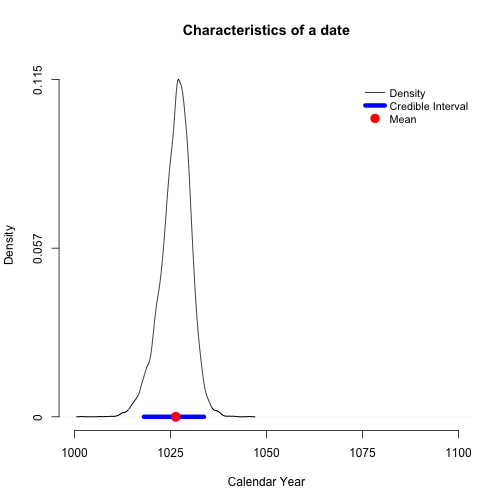The date (mean posterior distribution) of the sunspot, estimated using a simple Bayesian model that combines dates and allows for individual random effects, is dates at 1026 years after Christ. This date is associated with a 95% confidence interval : [1018, 1034].